Quamtum Computing                                                                        Home : www.sharetechnote.com Bloch Sphere   In most of simple operations or representations, I used the qbit state circle as shown here. It is very simple and easy to understand, but the Qbit state circle has restrictions. Since it is only in 2 dimension, it cannot represent the result of operation that requires 3 D representation. On top of it, it cannot represent the state function that has imaginary probability compoment. There is another way of visualizing a qbit that can overcome all the restrictions mentioned above. It is called Bloch Sphere which is named after the person (Felix Bloch) who invented the concept.   Simply put, Bloch Sphere is a way to respresent a qbit states in a 3D spherical coordinates. As you know, Spherical Coordinates is a system defined by R (radius), theta and phi. In Bloch sphere, R is fixed to 1.  Only Phi and Theta are vairable. The qbit state function in the Bloch Sphere is defined as follows.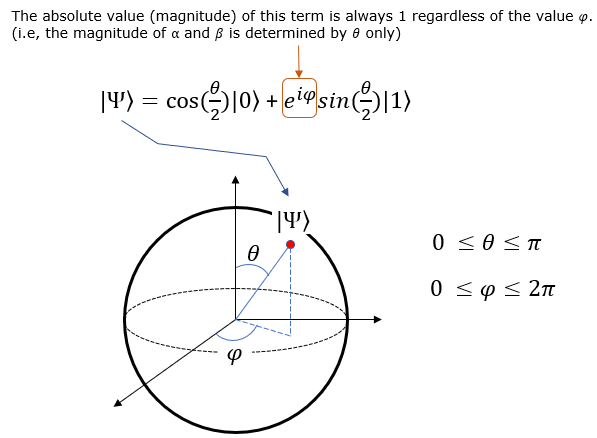Even though the qbit state function on Bloch sphere looks a little bit complicated, it is still in the form of probability for two qbit state |0> and |1>.  The probability of |0> and |1> (alpha and beta) is transformed into a little bit complicated form in Bloch sphere as shown below. Here you would notice that the probability of |1> can be expressed in complex number.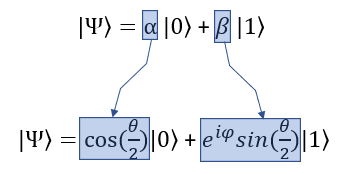Bloch Vectors on each Axis   Now for practice, let's think of some of the important points in the coordinate and how it is expressed in state function.   Let's take the point on the circumference that crosses the +z axis. In spherical coordinate, this point is where theta = 0 and phi = 0. If you plug these values into Bloch sphere state function you will get |0> as shown below.Now Let's take the point on the circumference that crosses the -z axis. In spherical coordinate, this point is where theta = pi and phi = 0. If you plug these values into Bloch sphere state function you will get |1> as shown below.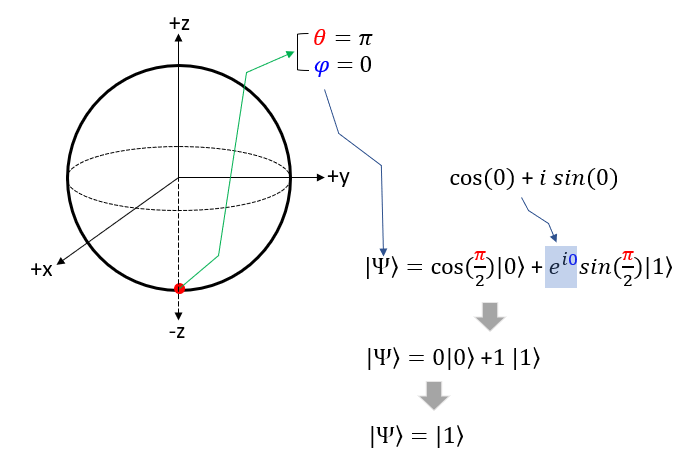Now Let's take the point on the circumference that crosses the -z axis. In spherical coordinate, this point is where theta = pi/2 and phi = 0. If you plug these values into Bloch sphere state function you will get the function with the same proability of |0> and |1> as shown below. In many documents, you would see this function is represented to be |+> for simplicity.Now Let's take the point on the circumference that crosses the -x axis. In spherical coordinate, this point is where theta = pi/2 and phi = pi. If you plug these values into Bloch sphere state function you will get the function with the same proability of |0> and |1> as shown below. In many documents, you would see this function is represented to be |-> for simplicity.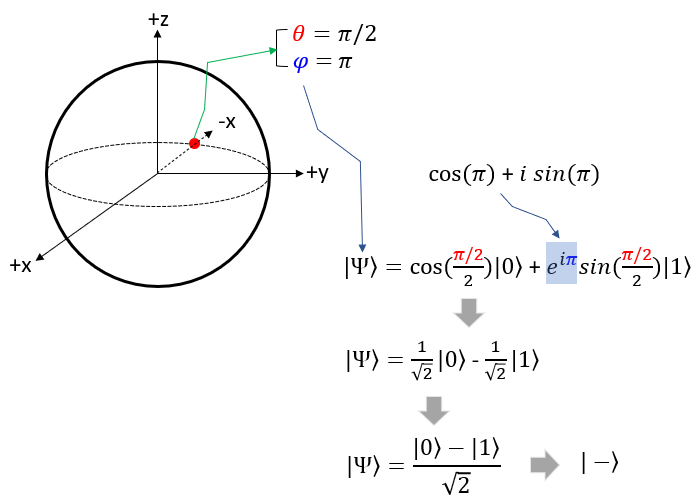Now Let's take the point on the circumference that crosses the +y axis. In spherical coordinate, this point is where theta = pi/2 and phi = pi. If you plug these values into Bloch sphere state function you will get the function with the same proability of |0> and |1> , but the probability of |1> is imaginary number as shown below. In many documents, you would see this function is represented to be |+i> for simplicity.Now Let's take the point on the circumference that crosses the -y axis. In spherical coordinate, this point is where theta = pi/2 and phi = pi. If you plug these values into Bloch sphere state function you will get the function with the same proability of |0> and |1> , but the probability of |1> is imaginary number as shown below. In many documents, you would see this function is represented to be |-i> for simplicity.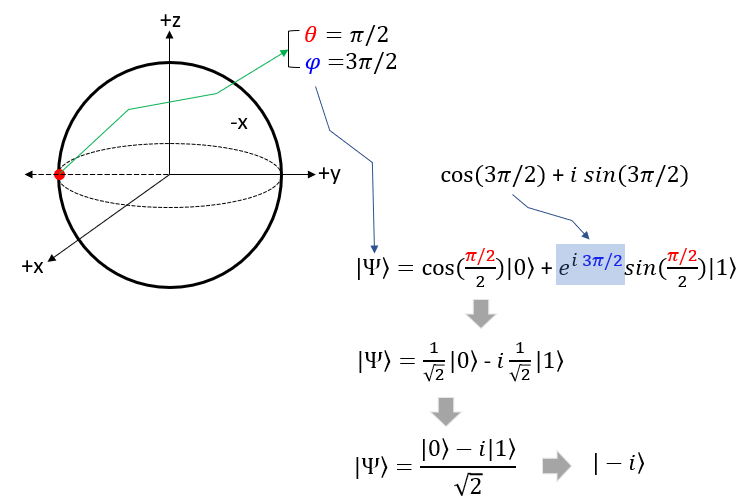Putting All Together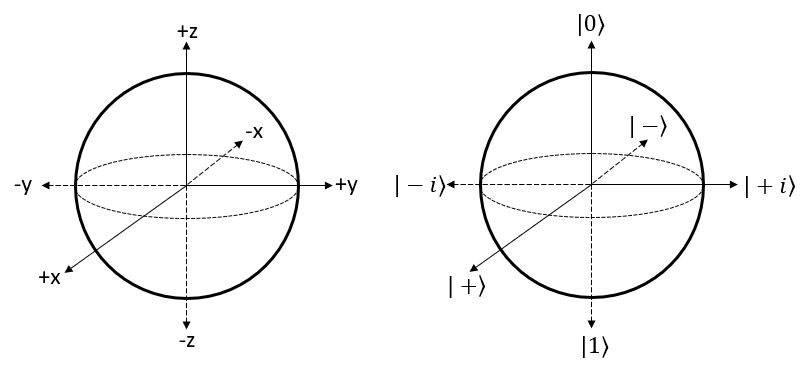Reference :